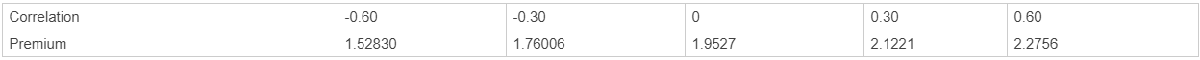Price European basket options using Nengjiu Ju approximation model

## Syntax

``Price = basketbyju(RateSpec,BasketStockSpec,OptSpec,Strike,Settle,Maturity)``

## Description

example

````Price = basketbyju(RateSpec,BasketStockSpec,OptSpec,Strike,Settle,Maturity)` prices European basket options using the Nengjiu Ju approximation model.```

## Examples

collapse all

Find a European call basket option of two stocks. Assume that the stocks are currently trading at \$10 and \$11.50 with annual volatilities of 20% and 25%, respectively. The basket contains one unit of the first stock and one unit of the second stock. The correlation between the assets is 30%. On January 1, 2009, an investor wants to buy a 1-year call option with a strike price of \$21.50. The current annualized, continuously compounded interest rate is 5%. Use this data to compute the price of the call basket option with the Nengjiu Ju approximation model.

```Settle = 'Jan-1-2009'; Maturity = 'Jan-1-2010'; % Define the RateSpec. Rate = 0.05; Compounding = -1; RateSpec = intenvset('ValuationDate', Settle, 'StartDates', ... Settle, 'EndDates', Maturity, 'Rates', Rate, 'Compounding', Compounding); % Define the Correlation matrix. Correlation matrices are symmetric and % have ones along the main diagonal. Corr = [1 0.30; 0.30 1]; % Define the BasketStockSpec. AssetPrice = [10;11.50]; Volatility = [0.2;0.25]; Quantity = [1;1]; BasketStockSpec = basketstockspec(Volatility, AssetPrice, Quantity, Corr); % Compute the price of the call basket option. OptSpec = {'call'}; Strike = 21.5; PriceCorr30 = basketbyju(RateSpec, BasketStockSpec, OptSpec, Strike, Settle, Maturity)```
```PriceCorr30 = 2.1221 ```

Compute the price of the basket instrument for these two stocks with a correlation of 60%. Then compare this cost to the total cost of buying two individual call options.

```Corr = [1 0.60; 0.60 1]; % Define the new BasketStockSpec. BasketStockSpec = basketstockspec(Volatility, AssetPrice, Quantity, Corr); % Compute the price of the call basket option with Correlation = -0.60 PriceCorr60 = basketbyju(RateSpec, BasketStockSpec, OptSpec, Strike, Settle, Maturity)```
```PriceCorr60 = 2.2757 ```

The following table summarizes the sensitivity of the option to correlation changes. In general, the premium of the basket option decreases with lower correlation and increases with higher correlation.Compute the cost of two vanilla 1-year call options using the Black-Scholes (BLS) model on the individual assets:

```StockSpec = stockspec(Volatility, AssetPrice); StrikeVanilla= [10;11.5]; PriceVanillaOption = optstockbybls(RateSpec, StockSpec, Settle, Maturity,... OptSpec, StrikeVanilla)```
```PriceVanillaOption = 2×1 1.0451 1.4186 ```

Find the total cost of buying two individual call options.

`sum(PriceVanillaOption) `
```ans = 2.4637 ```

The total cost of purchasing two individual call options is \$2.4637, compared to the maximum cost of the basket option of \$2.27 with a correlation of 60%.

## Input Arguments

collapse all

Interest-rate term structure (annualized and continuously compounded), specified by the `RateSpec` obtained from `intenvset`. For information on the interest-rate specification, see `intenvset`.

Data Types: `struct`

`BasketStock` specification, specified using `basketstockspec`.

Data Types: `struct`

Definition of the option as `'call'` or `'put'`, specified as a character vector or a `2`-by-`1` cell array of character vectors.

Data Types: `char` | `cell`

Option strike price value, specified as one of the following:

• For a European or Bermuda option, `Strike` is a scalar (European) or `1`-by-`NSTRIKES` (Bermuda) vector of strike prices.

• For an American option, `Strike` is a scalar vector of the strike price.

Data Types: `double`

Settlement or trade date for the basket option, specified as a scalar serial date number or date character vector.

Data Types: `double` | `char`

Maturity date for the basket option, specified as a scalar serial date number or date character vector.

Data Types: `double` | `char`

## Output Arguments

collapse all

Expected price for basket option, returned as a numeric.

collapse all